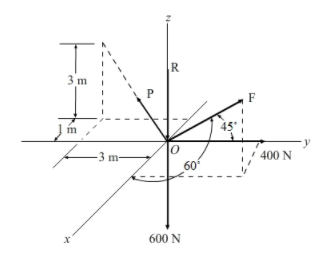# Problem: Determine the magnitudes of the forces P, R, F required for equilibrium of point O.A. R = 238 N, F = 181.0 N, P = 395 NB. R = 1340 N, F = 740 N, P = 538 NC. R = 419 N, F = 181.0 N, P = 395 ND. R = 408 N, F = 504 N, P = 1099 N

###### FREE Expert Solution

The equilibrium condition:

$\overline{){\mathbf{\sum }}{\mathbf{F}}{\mathbf{=}}{\mathbf{0}}}$

Let's start by expressing the forces P in Cartesian vector form:

$\begin{array}{rcl}\mathbf{P}& \mathbf{=}& \mathrm{P}\mathbf{\left[}\frac{\mathbf{-}\mathbf{i}\mathbf{-}\mathbf{3}\mathbf{j}\mathbf{+}\mathbf{3}\mathbf{k}}{\sqrt{{\mathbf{\left(}\mathbf{-}\mathbf{1}\mathbf{\right)}}^{\mathbf{2}}\mathbf{+}{\mathbf{\left(}\mathbf{-}\mathbf{3}\mathbf{\right)}}^{\mathbf{2}}\mathbf{+}{\mathbf{3}}^{\mathbf{2}}}}\mathbf{\right]}\\ & =& \mathrm{P}\mathbf{\left[}\frac{\mathbf{-}\mathbf{i}\mathbf{-}\mathbf{3}\mathbf{j}\mathbf{+}\mathbf{3}\mathbf{k}}{\sqrt{\mathbf{19}}}\mathbf{\right]}\end{array}$

94% (205 ratings)###### Problem DetailsDetermine the magnitudes of the forces P, R, F required for equilibrium of point O.

A. R = 238 N, F = 181.0 N, P = 395 N

B. R = 1340 N, F = 740 N, P = 538 N

C. R = 419 N, F = 181.0 N, P = 395 N

D. R = 408 N, F = 504 N, P = 1099 N